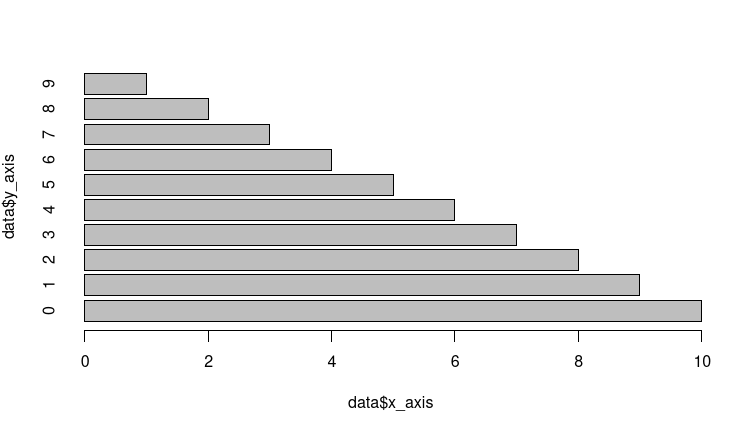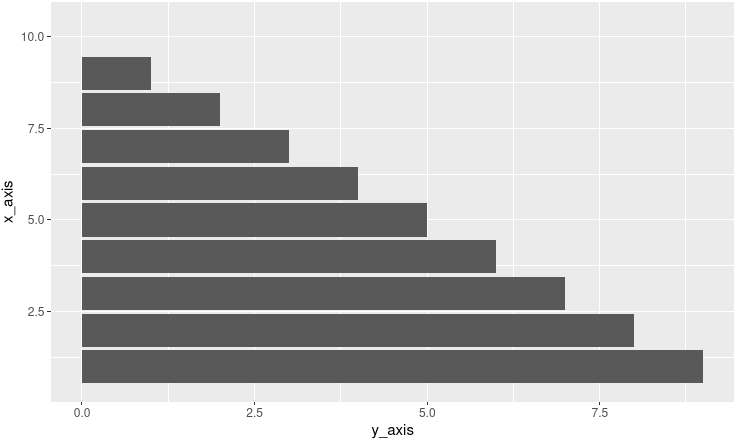# How to Draw a Horizontal Barplot in R

• Last Updated : 03 Dec, 2021

A barplot is a representation of data in form of the bar the height of bar represents the number of values. In this article, we will discuss How to Draw a Horizontal Barplot in R programming language.

R language supports two ways to plot a bar plot, and we will discuss how this can be modified to be put to our use.

### Method 1: Using barplot()

R Language uses the function barplot() to create bar charts. Here, both vertical and Horizontal bars can be drawn.

Syntax: barplot(H, xlab, ylab, main, names.arg, col)

Parameters:

• H: This parameter is a vector or matrix containing numeric values which are used in bar chart.
• xlab: This parameter is the label for x axis in bar chart.
• ylab: This parameter is the label for y axis in bar chart.
• main: This parameter is the title of the bar chart.
• names.arg: This parameter is a vector of names appearing under each bar in bar chart.
• col: This parameter is used to give colors to the bars in the graph.

The bar function has a field named “horiz” keep that field value “True” to create the bar plot horizontally.

Example:

## R

 `# Create example data``data <- ``data.frame``(x_axis = (1:10),  ``                   ``y_axis = 9:0)` `# barplot() function is used to``# plot the bar and horiz field is``# used to plot bar horizontally``barplot``(data\$x_axis ~ data\$y_axis,``        ``horiz = ``TRUE``)`

Output:fig 1: Horizontal bar plot

### Method 2: Using GGPLOT2

In this method, we draw the bar plot using the ggplot2 function. ggplot2 have a function named geom_bar() which is used to plot the horizontal bar, and we put our data into the geom_bar function with ggplot() to plot the bar. At last, we will flip the whole plot using the coord_flip() function. A flipped vertical plot will generate a horizontal plot.

Example:

## R

 `# load the library              ``library``(``"ggplot2"``)` `# data frame created``data <- ``data.frame``(x_axis = (1:10),  ``                   ``y_axis = 9:0)` `# bar is created with the help of``# grom_bar() and ggplot() function``ggp <- ``ggplot``(data, ``aes``(x_axis, y_axis)) +   ``  ``geom_bar``(stat = ``"identity"``)` `# complete graph get flipped with the``# help of coord_flip() function``ggp +  ``coord_flip``()`

Output:fig 2: Horizontal bar plot

My Personal Notes arrow_drop_up